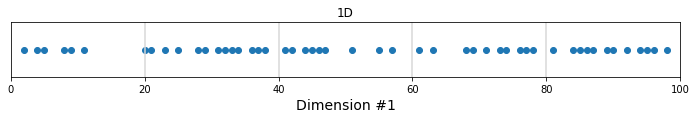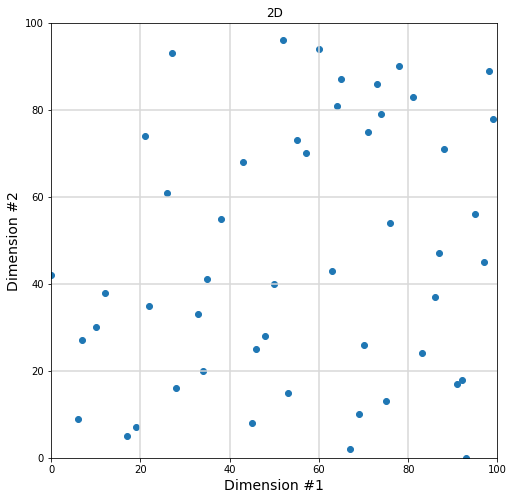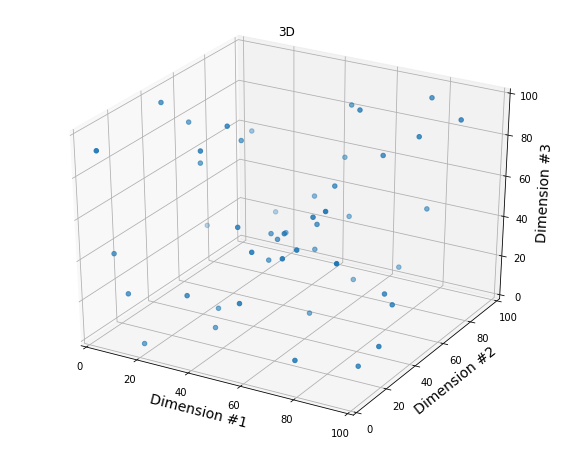# The Curse of Dimensionality – Illustrated With Matplotlib

Maybe you already came across this famous quote in Machine Learning by Charles Lee Isbell

“As the number of features or dimensions grows, the amount of data we need to generalize accurately grows exponentially.”

Here is another explanation from Wikipedia

“When the dimensionality increases, the volume of the space increases so fast that the available data become sparse. (…) In order to obtain a statistically sound and reliable result, the amount of data needed to support the result often grows exponentially with the dimensionality.”

I think the  “Curse of Dimensionality” is easier to understand when visualized. Suppose you have 50 data points between 0 and 100.

### 1- Let’s try with one dimension first

```import pandas as pd
import matplotlib.pyplot as plt
import random
import numpy as np

fig = plt.figure()
ax  = plt.axes()
fig.set_size_inches(12, 1)

x = random.sample(range(0, 100), 50)
y = [0 for xval in x]
plt.scatter(x, y)

# Grid lines
for grid_pt in [20, 40, 60, 80]:
plt.axvline(x=grid_pt, color='#D8D8D8')

ax.set_xlim((0,100))
ax.set_xlabel("Dimension #1", fontsize=14)
ax.set_ylabel("")
plt.yticks([], [])
plt.title("1D")
plt.show()```With 5 intervals in our first dimension, there will be an average of 50/5 = 10 points per cell, which is already low if you’d like to do any statistical analysis for each interval.

### 2- Moving to two dimensions

```fig = plt.figure()
ax  = plt.axes()
fig.set_size_inches(8, 8)

# Now each point has 2 dimensions (x,y)
x = random.sample(range(0, 100), 50)
y = random.sample(range(0, 100), 50)

plt.scatter(x, y)

# Grid lines
for grid_pt in [20, 40, 60, 80]:
plt.axvline(x=grid_pt, color='#D8D8D8')
plt.axhline(y=grid_pt, color='#D8D8D8')

ax.set_xlim((0,100))
ax.set_ylim((0,100))
ax.set_xlabel("Dimension #1", fontsize=14)
ax.set_ylabel("Dimension #2", fontsize=14)
plt.title("2D")
plt.show()```With 5 intervals on the first dimension and 5 intervals on the 2nd dimension, we now have 50/(5×5) = 2 points per cell on average. In fact, we are already starting to see cells that do not have any data to work with.

### 3- Adding a third dimension

```from mpl_toolkits import mplot3d

fig = plt.figure()
fig.set_size_inches(10, 8)

# Now each point has 3 dimensions (x,y,x)
x = random.sample(range(0, 100), 50)
y = random.sample(range(0, 100), 50)
z = random.sample(range(0, 100), 50)

ax.scatter(x, y, z)

# Grid lines
for grid_pt in [20, 40, 60, 80]:
plt.axvline(x=grid_pt, color='#D8D8D8')
plt.axhline(y=grid_pt, color='#D8D8D8')

ax.set_xlim(0,100)
ax.set_ylim(0,100)
ax.set_zlim(0,100)

ax.set_xlabel("Dimension #1", fontsize=14)
ax.set_ylabel("Dimension #2", fontsize=14)
ax.set_zlabel("Dimension #3", fontsize=14)
plt.title("3D")
plt.show()```With 5 intervals on the third dimension, we have 50/(5x5x5) = 0.4 points per cell on average!

### In Conclusion

As you add new dimensions, you create “new space” that is usually not filled properly by your initial data.

In order to obtain a statistically sound and reliable result, the amount of data needed to support the result often grows exponentially with the dimensionality.